Q-Chem 5.0 User’s Manual

# 4.5 SCF Initial Guess

## 4.5.1 Introduction

The Roothaan-Hall and Pople-Nesbet equations of SCF theory are non-linear in the molecular orbital coefficients. Like many mathematical problems involving non-linear equations, prior to the application of a technique to search for a numerical solution, an initial guess for the solution must be generated. If the guess is poor, the iterative procedure applied to determine the numerical solutions may converge very slowly, requiring a large number of iterations, or at worst, the procedure may diverge.

Thus, in an ab initio SCF procedure, the quality of the initial guess is of utmost importance for (at least) two main reasons:

• To ensure that the SCF converges to an appropriate ground state. Often SCF calculations can converge to different local minima in wave function space, depending upon which part of “LCAO space” in which the initial guess lands.

• When considering jobs with many basis functions requiring the recalculation of ERIs at each iteration, using a good initial guess that is close to the final solution can reduce the total job time significantly by decreasing the number of SCF iterations.

For these reasons, sooner or later most users will find it helpful to have some understanding of the different options available for customizing the initial guess. Q-Chem currently offers six options for the initial guess:

• Superposition of Atomic Density (SAD)

• Core Hamiltonian (CORE)

• Generalized Wolfsberg-Helmholtz (GWH)

• Basis set projection (BASIS2)

The first four of these guesses are built-in, and are briefly described in Section 4.5.2. The option of reading MOs from disk is described in Section 4.5.3. The initial guess MOs can be modified, either by mixing, or altering the order of occupation. These options are discussed in Section 4.5.4. Finally, Q-Chem’s novel basis set projection method is discussed in Section 4.5.5.

## 4.5.2 Simple Initial Guesses

There are four simple initial guesses available in Q-Chem. While they are all simple, they are by no means equal in quality, as we discuss below.

1. Superposition of Atomic Densities (SAD): The SAD guess is almost trivially constructed by summing together atomic densities that have been spherically averaged to yield a trial density matrix. The SAD guess is far superior to the other two options below, particularly when large basis sets and/or large molecules are employed. There are three issues associated with the SAD guess to be aware of:

1. No molecular orbitals are obtained, which means that SCF algorithms requiring orbitals (the direct minimization methods discussed in Section 4.6) cannot directly use the SAD guess, and,

2. The SAD guess is not available for general (read-in) basis sets. All internal basis sets support the SAD guess.

3. The SAD guess is not idempotent and thus requires at least two SCF iterations to ensure proper SCF convergence (idempotency of the density).

2. Purified Superposition of Atomic Densities (SADMO): This guess is similar to the SAD guess, with two critical differences, namely, the removal of issues 1 and 3 above. The functional difference to the SAD guess is that the density matrix obtained from the superposition is diagonalized to obtain natural molecular orbitals, after which an idempotent density matrix is created by aufbau occupation of the natural orbitals. Since the initial density matrix is created with the SAD guess, the SADMO guess is not available either for a general (read-in) basis set.

3. Generalized Wolfsberg-Helmholtz (GWH): The GWH guess procedure  uses a combination of the overlap matrix elements in Eq. (4.12), and the diagonal elements of the Core Hamiltonian matrix in Eq. (4.20). This initial guess is most satisfactory in small basis sets for small molecules. It is constructed according to the relation given below, where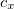is a constant typically chosen as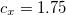.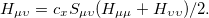(4.80)
4. Core Hamiltonian: The core Hamiltonian guess simply obtains the guess MO coefficients by diagonalizing the core Hamiltonian matrix in Eq. (4.20). This approach works best with small basis sets, and degrades as both the molecule size and the basis set size are increased.

The selection of these choices (or whether to read in the orbitals) is controlled by the following $rem variables: SCF_GUESS  Specifies the initial guess procedure to use for the SCF. TYPE:  STRING DEFAULT:  SAD Superposition of atomic densities (available only with standard basis sets) GWH For ROHF where a set of orbitals are required. FRAGMO For a fragment MO calculation OPTIONS:  CORE Diagonalize core Hamiltonian SAD Superposition of atomic density SADMO Purified superposition of atomic densities (available only with standard basis sets) GWH Apply generalized Wolfsberg-Helmholtz approximation READ Read previous MOs from disk FRAGMO Superimposing converged fragment MOs RECOMMENDATION:  SAD or SADMO guess for standard basis sets. For general basis sets, it is best to use the BASIS2$rem. Alternatively, try the GWH or core Hamiltonian guess. For ROHF it can be useful to READ guesses from an SCF calculation on the corresponding cation or anion. Note that because the density is made spherical, this may favor an undesired state for atomic systems, especially transition metals. Use FRAGMO in a fragment MO calculation.

SCF_GUESS_ALWAYS
 Switch to force the regeneration of a new initial guess for each series of SCF iterations (for use in geometry optimization).

TYPE:
 LOGICAL

DEFAULT:
 False

OPTIONS:
 False Do not generate a new guess for each series of SCF iterations in an optimization; use MOs from the previous SCF calculation for the guess, if available. True Generate a new guess for each series of SCF iterations in a geometry optimization.

RECOMMENDATION:
 Use the default unless SCF convergence issues arise

## 4.5.3 Reading MOs from Disk

There are two methods by which MO coefficients can be used from a previous job by reading them from disk:

1. Running two independent jobs sequentially invoking qchem with three command line variables:.

   localhost-1> qchem job1.in job1.out save
localhost-2> qchem job2.in job2.out save


Note: (1) The $rem variable SCF_GUESS must be set to READ in job2.in. (2) Scratch files remain in$QCSCRATCH/save on exit.

2. Running a batch job where two jobs are placed into a single input file separated by the string @@@ on a single line.
Note: (1) SCF_GUESS must be set to READ in the second job of the batch file.
(2) A third qchem command line variable is not necessary.
(3) As for the SAD guess, Q-Chem requires at least two SCF cycles to ensure proper
SCF convergence (idempotency of the density).

Note: It is up to the user to make sure that the basis sets match between the two jobs. There is no internal checking for this, although the occupied orbitals are re-orthogonalized in the current basis after being read in. If you want to project from a smaller basis into a larger basis, consult section 4.5.5.

## 4.5.4 Modifying the Occupied Molecular Orbitals

It is sometimes useful for the occupied guess orbitals to be other than the lowest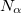(or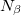) orbitals. Reasons why one may need to do this include:

• To converge to a state of different symmetry or orbital occupation.

• To break spatial symmetry.

• To break spin symmetry, as in unrestricted calculations on molecules with an even number of electrons.

There are two mechanisms for modifying a set of guess orbitals: either by SCF_GUESS_MIX, or by specifying the orbitals to occupy. Q-Chem users may define the occupied guess orbitals using the $occupied or$swap_occupied_virtual keywords. In the former, occupied guess orbitals are defined by listing the alpha orbitals to be occupied on the first line and beta on the second. In the former, only pair of orbitals that needs to be swapped is specified.

Note: To prevent Q-Chem to change orbital occupation during SCF procedure, MOM_START option is often used in combination with $occupied or$swap_occupied_virtual keywords.

Note: The need for orbitals renders these options incompatible with the SAD guess. Most often, they are used with SCF_GUESS = READ.

Example 4.42  Format for modifying occupied guess orbitals.

$occupied 1 2 3 4 ... nalpha 1 2 3 4 ... nbeta$end


Example 4.43  Alternative format for modifying occupied guess orbitals.

$swap_occupied_virtual <spin> <io1> <iv1> <spin> <io2> <iv2>$end


Example 4.44  Example of swapping guess orbitals.

$swap_occupied_virtual alpha 5 6 beta 6 7$end


This is identical to: Example 4.45  Example of specifying occupied guess orbitals.

$occupied 1 2 3 4 6 5 7 1 2 3 4 5 7 6$end


or Example 4.46  Example of specifying occupied guess orbitals.

$occupied 1:4 6 5 7 1:5 7 6$end


The other $rem variables related to altering the orbital occupancies are: SCF_GUESS_PRINT  Controls printing of guess MOs, Fock and density matrices. TYPE:  INTEGER DEFAULT:  0 OPTIONS:  0 Do not print guesses. SAD 1 Atomic density matrices and molecular matrix. 2 Level 1 plus density matrices. CORE and GWH 1 No extra output. 2 Level 1 plus Fock and density matrices and, MO coefficients and eigenvalues. READ 1 No extra output 2 Level 1 plus density matrices, MO coefficients and eigenvalues. RECOMMENDATION:  None SCF_GUESS_MIX  Controls mixing of LUMO and HOMO to break symmetry in the initial guess. For unrestricted jobs, the mixing is performed only for the alpha orbitals. TYPE:  INTEGER DEFAULT:  0 (FALSE) Do not mix HOMO and LUMO in SCF guess. OPTIONS:  0 (FALSE) Do not mix HOMO and LUMO in SCF guess. 1 (TRUE) Add 10% of LUMO to HOMO to break symmetry.Addof LUMO to HOMO (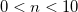). RECOMMENDATION:  When performing unrestricted calculations on molecules with an even number of electrons, it is often necessary to break alpha/beta symmetry in the initial guess with this option, or by specifying input for$occupied.

## 4.5.5 Basis Set Projection

Q-Chem also includes a novel basis set projection method developed by Dr Jing Kong of Q-Chem Inc. It permits a calculation in a large basis set to bootstrap itself up via a calculation in a small basis set that is automatically spawned when the user requests this option. When basis set projection is requested (by providing a valid small basis for BASIS2), the program executes the following steps:

• A simple DFT calculation is performed in the small basis, BASIS2, yielding a converged density matrix in this basis.

• The large basis set SCF calculation (with different values of EXCHANGE and CORRELATION set by the input) begins by constructing the DFT Fock operator in the large basis but with the density matrix obtained from the small basis set.

• By diagonalizing this matrix, an accurate initial guess for the density matrix in the large basis is obtained, and the target SCF calculation commences.

Two different methods of projection are available and can be set using the BASISPROJTYPE $rem. The OVPROJECTION option expands the MOs from the BASIS2 calculation in the larger basis, while the FOPPROJECTION option constructs the Fock matrix in the larger basis using the density matrix from the initial, smaller basis set calculation. Basis set projection is a very effective option for general basis sets, where the SAD guess is not available. In detail, this initial guess is controlled by the following$rem variables:

BASIS2
 Sets the small basis set to use in basis set projection.

TYPE:
 STRING

DEFAULT:
 No second basis set default.

OPTIONS:
 Symbol. Use standard basis sets as per Chapter 7. BASIS2_GEN General BASIS2 BASIS2_MIXED Mixed BASIS2

RECOMMENDATION:
 BASIS2 should be smaller than BASIS. There is little advantage to using a basis larger than a minimal basis when BASIS2 is used for initial guess purposes. Larger, standardized BASIS2 options are available for dual-basis calculations (see Section 4.9).

BASISPROJTYPE
 Determines which method to use when projecting the density matrix of BASIS2

TYPE:
 STRING

DEFAULT:
 FOPPROJECTION (when DUAL_BASIS_ENERGY=false) OVPROJECTION (when DUAL_BASIS_ENERGY=true)

OPTIONS:
 FOPPROJECTION Construct the Fock matrix in the second basis OVPROJECTION Projects MOs from BASIS2 to BASIS.

RECOMMENDATION:
 None

Note: BASIS2 sometimes affects post-Hartree-Fock calculations. It is recommended to split such jobs into two subsequent one, such that in the first job a desired Hartree-Fock solution is found using BASIS2, and in the second job, which performs a post-HF calculation, SCF_GUESS=READ is invoked.

## 4.5.6 Examples

Example 4.47  Input where basis set projection is used to generate a good initial guess for a calculation employing a general basis set, for which the default initial guess is not available.

$molecule 0 1 O H 1 r H 1 r 2 a r 0.9 a 104.0$end

$rem METHOD mp2 BASIS general BASIS2 sto-3g$end

$basis O 0 S 3 1.000000 3.22037000E+02 5.92394000E-02 4.84308000E+01 3.51500000E-01 1.04206000E+01 7.07658000E-01 SP 2 1.000000 7.40294000E+00 -4.04453000E-01 2.44586000E-01 1.57620000E+00 1.22156000E+00 8.53955000E-01 SP 1 1.000000 3.73684000E-01 1.00000000E+00 1.00000000E+00 SP 1 1.000000 8.45000000E-02 1.00000000E+00 1.00000000E+00 **** H 0 S 2 1.000000 5.44717800E+00 1.56285000E-01 8.24547000E-01 9.04691000E-01 S 1 1.000000 1.83192000E-01 1.00000000E+00 ****$end


Example 4.48  Input for an ROHF calculation on the OH radical. One SCF cycle is initially performed on the cation, to get reasonably good initial guess orbitals, which are then read in as the guess for the radical. This avoids the use of Q-Chem’s default GWH guess for ROHF, which is often poor.

$comment OH radical, part 1. Do 1 iteration of cation orbitals.$end

$molecule 1 1 O 0.000 0.000 0.000 H 0.000 0.000 1.000$end

$rem BASIS = 6-311++G(2df) METHOD = hf MAX_SCF_CYCLES = 1 THRESH = 10$end

@@@

$comment OH radical, part 2. Read cation orbitals, do the radical$end

$molecule 0 2 O 0.000 0.000 0.000 H 0.000 0.000 1.000$end

$rem BASIS = 6-311++G(2df) METHOD = hf UNRESTRICTED = false SCF_ALGORITHM = dm SCF_CONVERGENCE = 7 SCF_GUESS = read THRESH = 10$end


Example 4.49  Input for an unrestricted HF calculation on Hin the dissociation limit, showing the use of SCF_GUESS_MIX = 2 (corresponding to 20% of the alpha LUMO mixed with the alpha HOMO). Geometric direct minimization with DIIS is used to converge the SCF, together with MAX_DIIS_CYCLES = 1 (using the default value for MAX_DIIS_CYCLES, the DIIS procedure just oscillates).

$molecule 0 1 H 0.000 0.000 0.0 H 0.000 0.000 -10.0$end

$rem UNRESTRICTED = true METHOD = hf BASIS = 6-31g** SCF_ALGORITHM = diis_gdm MAX_DIIS_CYCLES = 1 SCF_GUESS = gwh SCF_GUESS_MIX = 2$end